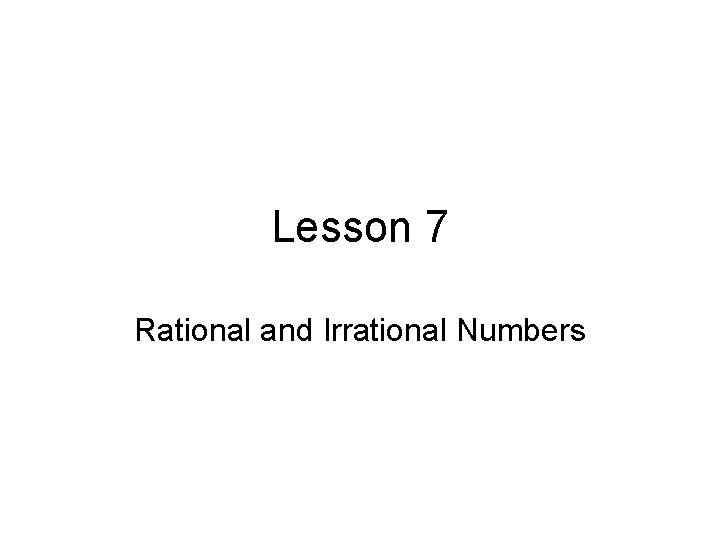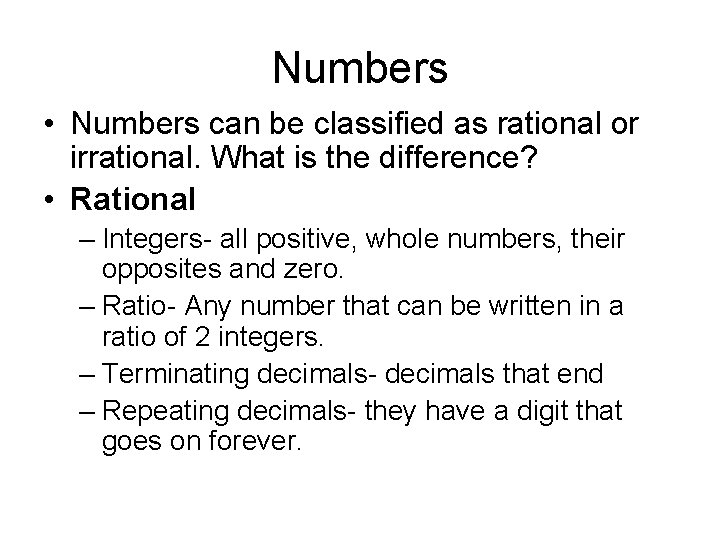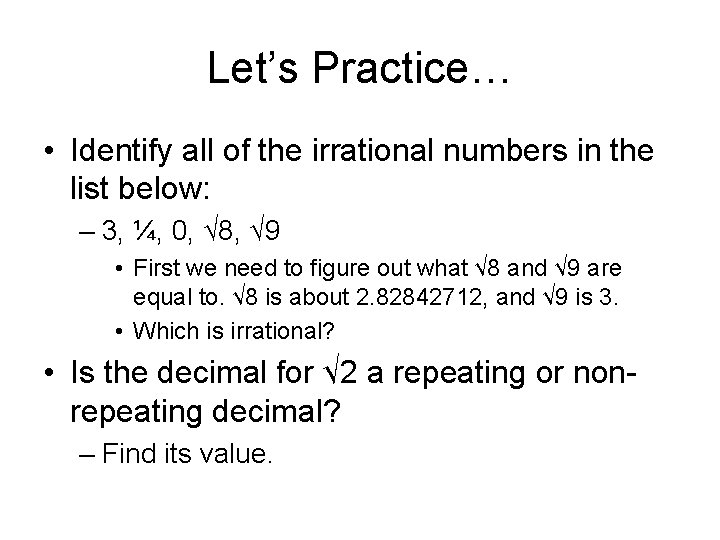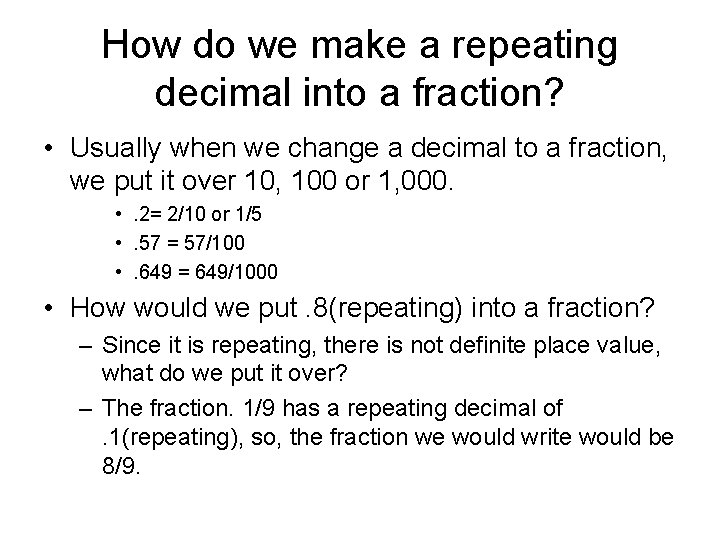# Lesson 7 Rational and Irrational Numbers Numbers Numbers

• Slides: 7Lesson 7 Rational and Irrational NumbersNumbers • Numbers can be classified as rational or irrational. What is the difference? • Rational – Integers- all positive, whole numbers, their opposites and zero. – Ratio- Any number that can be written in a ratio of 2 integers. – Terminating decimals- decimals that end – Repeating decimals- they have a digit that goes on forever.Then what’s irrational? • If a number can’t be written as the ratio of two integers, it is irrational. • All non-terminating, non-repeating decimals are irrational. – 3. 14159265… we know this as pi. It is a non-repeating decimal. Its digits go on forever, but never repeat. – 1. 7320508…is irrational because when written as a decimal its digits never end and never repeat. • There is no way to write non-terminating decimals as a ratio.Let’s Practice… • Identify all of the irrational numbers in the list below: – 3, ¼, 0, √ 8, √ 9 • First we need to figure out what √ 8 and √ 9 are equal to. √ 8 is about 2. 82842712, and √ 9 is 3. • Which is irrational? • Is the decimal for √ 2 a repeating or nonrepeating decimal? – Find its value.How do we make a repeating decimal into a fraction? • Usually when we change a decimal to a fraction, we put it over 10, 100 or 1, 000. • . 2= 2/10 or 1/5 • . 57 = 57/100 • . 649 = 649/1000 • How would we put. 8(repeating) into a fraction? – Since it is repeating, there is not definite place value, what do we put it over? – The fraction. 1/9 has a repeating decimal of. 1(repeating), so, the fraction we would write would be 8/9.Practice time! • What decimal represents √ 5? • What is the decimal equivalent to 4/9? • Which number is a rational number? 0. 76, 0. 83961257…, √ 10, √ 14 • What decimal is equivalent to 2/3? • An irrational number is….Last but not least • Look at the list of numbers below. Π, -0. 005, -9/7, √ 12, √ 81 – Name two different irrational numbers in the list above. – Explain how you know that each number you chose is irrational.